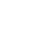• 支持90多种语言
我们可以将任何类型的文件翻译成 90 多种语言。
• 最优价格保证
我们能与网上高评价的竞争对手比价。
• 可选当日交稿
当您选择我们的标准头等速度时，大多数的文件将在 1-2 个工作日内送达。
为保证能当日交稿，请在下订单时选择加急翻译服务

Service and Speed Unaffected by COVID-19

Delivery timings for both urgent and standard orders are unaffected by the COVID-19 pandemic. The Spanish Group has medical translators on staff to expedite COVID-19 related translations.

Get Started1. 文件语言

• 中文（繁体中文）
• 英语
• 法语
• 西班牙语
• 中文（简体中文）
• 意大利语
• 葡萄牙语（欧洲）
• 葡萄牙语（巴西）
• 韩语
• 日语
• 阿拉伯语
• 俄罗斯语
• 德语
• 中文（简体中文）
• 中文（繁体中文）
• 丹麦语
• 乌克兰语
• 乌兹别克语
• 乌尔都语
• 亚美尼亚语
• 他加禄语（菲律宾）
• 俄罗斯语
• 保加利亚语
• 信德语
• 僧伽罗语
• 克伦语支
• 克罗地亚语
• 冰岛语
• 加利西亚语
• 加泰罗尼亚语
• 匈牙利语
• 南非语
• 卡纳达语
• 卢森堡语
• 印地语
• 印尼语
• 印尼语
• 古吉拉特语
• 吉尔吉斯语
• 哈萨克语
• 土耳其语
• 塔吉克语
• 塞尔维亚语
• 塞索托语
• 奇契瓦语
• 契维语
• 威尔士语
• 孟加拉语
• 宿务语
• 尼泊尔语
• 巴斯克语
• 巽他语
• 希伯来语
• 希腊语
• 广东话
• 库尔德语（Kurmanji）
• 弗拉芒语
• 弗里西亚语
• 德语
• 意大利语
• 意第绪语
• 拉丁语
• 拉脱维亚语
• 挪威语
• 捷克语
• 提格利亚语
• 斯洛伐克语
• 斯洛文尼亚文
• 斯瓦希里语
• 旁遮普语
• 日语
• 普什图语
• 柬埔寨语（高棉语）
• 格鲁吉亚语
• 毛利语
• 法语
• 波兰语
• 波斯尼亚语
• 波斯语
• 波斯语（波斯语）
• 泰卢固语
• 泰米尔语
• 泰语
• 海地克里奥尔语
• 爪哇语
• 爱尔兰语
• 爱沙尼亚语
• 瑞典语
• 白俄罗斯语
• 祖鲁族
• 科萨语
• 科西嘉语
• 立陶宛语
• 索马里语
• 约鲁巴语
• 绍纳语
• 缅甸语
• 罗马尼亚语
• 老挝语
• 芬兰语
• 苏格兰盖尔语
• 苗语
• 英语
• 荷兰语
• 菲律宾语（他加禄语）
• 萨摩亚语
• 葡萄牙语（巴西）
• 葡萄牙语（欧洲）
• 蒙古语
• 西班牙语
• 豪萨语
• 越南语
• 达里语
• 阿塞拜疆语
• 阿姆哈拉语
• 阿尔巴尼亚语
• 阿拉伯语
• 韩语
• 马其顿语
• 马尔加什语
• 马拉塔语
• 马拉雅拉姆语
• 马来语
• 马耳他语
• 高棉语（柬埔寨语）
• 黑山语
2. 翻译语种

• 英语
• 中文（繁体中文）
• 法语
• 西班牙语
• 中文（简体中文）
• 意大利语
• 葡萄牙语（欧洲）
• 葡萄牙语（巴西）
• 韩语
• 日语
• 阿拉伯语
• 俄罗斯语
• 德语
• 中文（简体中文）
• 中文（繁体中文）
• 丹麦语
• 乌克兰语
• 乌兹别克语
• 乌尔都语
• 亚美尼亚语
• 他加禄语（菲律宾）
• 俄罗斯语
• 保加利亚语
• 信德语
• 僧伽罗语
• 克伦语支
• 克罗地亚语
• 冰岛语
• 加利西亚语
• 加泰罗尼亚语
• 匈牙利语
• 南非语
• 卡纳达语
• 卢森堡语
• 印地语
• 印尼语
• 印尼语
• 古吉拉特语
• 吉尔吉斯语
• 哈萨克语
• 土耳其语
• 塔吉克语
• 塞尔维亚语
• 塞索托语
• 奇契瓦语
• 契维语
• 威尔士语
• 孟加拉语
• 宿务语
• 尼泊尔语
• 巴斯克语
• 巽他语
• 希伯来语
• 希腊语
• 广东话
• 库尔德语（Kurmanji）
• 弗拉芒语
• 弗里西亚语
• 德语
• 意大利语
• 意第绪语
• 拉丁语
• 拉脱维亚语
• 挪威语
• 捷克语
• 提格利亚语
• 斯洛伐克语
• 斯洛文尼亚文
• 斯瓦希里语
• 旁遮普语
• 日语
• 普什图语
• 柬埔寨语（高棉语）
• 格鲁吉亚语
• 毛利语
• 法语
• 波兰语
• 波斯尼亚语
• 波斯语
• 波斯语（波斯语）
• 泰卢固语
• 泰米尔语
• 泰语
• 海地克里奥尔语
• 爪哇语
• 爱尔兰语
• 爱沙尼亚语
• 瑞典语
• 白俄罗斯语
• 祖鲁族
• 科萨语
• 科西嘉语
• 立陶宛语
• 索马里语
• 约鲁巴语
• 绍纳语
• 缅甸语
• 罗马尼亚语
• 老挝语
• 芬兰语
• 苏格兰盖尔语
• 苗语
• 英语
• 荷兰语
• 菲律宾语（他加禄语）
• 萨摩亚语
• 葡萄牙语（巴西）
• 葡萄牙语（欧洲）
• 蒙古语
• 西班牙语
• 豪萨语
• 越南语
• 达里语
• 阿塞拜疆语
• 阿姆哈拉语
• 阿尔巴尼亚语
• 阿拉伯语
• 韩语
• 马其顿语
• 马尔加什语
• 马拉塔语
• 马拉雅拉姆语
• 马来语
• 马耳他语
• 高棉语（柬埔寨语）
• 黑山语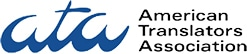1. 文件语言

• 中文（繁体中文）
• 英语
• 法语
• 西班牙语
• 中文（简体中文）
• 意大利语
• 葡萄牙语（欧洲）
• 葡萄牙语（巴西）
• 韩语
• 日语
• 阿拉伯语
• 俄罗斯语
• 德语
• 中文（简体中文）
• 中文（繁体中文）
• 丹麦语
• 乌克兰语
• 乌兹别克语
• 乌尔都语
• 亚美尼亚语
• 他加禄语（菲律宾）
• 俄罗斯语
• 保加利亚语
• 信德语
• 僧伽罗语
• 克伦语支
• 克罗地亚语
• 冰岛语
• 加利西亚语
• 加泰罗尼亚语
• 匈牙利语
• 南非语
• 卡纳达语
• 卢森堡语
• 印地语
• 印尼语
• 印尼语
• 古吉拉特语
• 吉尔吉斯语
• 哈萨克语
• 土耳其语
• 塔吉克语
• 塞尔维亚语
• 塞索托语
• 奇契瓦语
• 契维语
• 威尔士语
• 孟加拉语
• 宿务语
• 尼泊尔语
• 巴斯克语
• 巽他语
• 希伯来语
• 希腊语
• 广东话
• 库尔德语（Kurmanji）
• 弗拉芒语
• 弗里西亚语
• 德语
• 意大利语
• 意第绪语
• 拉丁语
• 拉脱维亚语
• 挪威语
• 捷克语
• 提格利亚语
• 斯洛伐克语
• 斯洛文尼亚文
• 斯瓦希里语
• 旁遮普语
• 日语
• 普什图语
• 柬埔寨语（高棉语）
• 格鲁吉亚语
• 毛利语
• 法语
• 波兰语
• 波斯尼亚语
• 波斯语
• 波斯语（波斯语）
• 泰卢固语
• 泰米尔语
• 泰语
• 海地克里奥尔语
• 爪哇语
• 爱尔兰语
• 爱沙尼亚语
• 瑞典语
• 白俄罗斯语
• 祖鲁族
• 科萨语
• 科西嘉语
• 立陶宛语
• 索马里语
• 约鲁巴语
• 绍纳语
• 缅甸语
• 罗马尼亚语
• 老挝语
• 芬兰语
• 苏格兰盖尔语
• 苗语
• 英语
• 荷兰语
• 菲律宾语（他加禄语）
• 萨摩亚语
• 葡萄牙语（巴西）
• 葡萄牙语（欧洲）
• 蒙古语
• 西班牙语
• 豪萨语
• 越南语
• 达里语
• 阿塞拜疆语
• 阿姆哈拉语
• 阿尔巴尼亚语
• 阿拉伯语
• 韩语
• 马其顿语
• 马尔加什语
• 马拉塔语
• 马拉雅拉姆语
• 马来语
• 马耳他语
• 高棉语（柬埔寨语）
• 黑山语
2. 翻译语种

• 英语
• 中文（繁体中文）
• 法语
• 西班牙语
• 中文（简体中文）
• 意大利语
• 葡萄牙语（欧洲）
• 葡萄牙语（巴西）
• 韩语
• 日语
• 阿拉伯语
• 俄罗斯语
• 德语
• 中文（简体中文）
• 中文（繁体中文）
• 丹麦语
• 乌克兰语
• 乌兹别克语
• 乌尔都语
• 亚美尼亚语
• 他加禄语（菲律宾）
• 俄罗斯语
• 保加利亚语
• 信德语
• 僧伽罗语
• 克伦语支
• 克罗地亚语
• 冰岛语
• 加利西亚语
• 加泰罗尼亚语
• 匈牙利语
• 南非语
• 卡纳达语
• 卢森堡语
• 印地语
• 印尼语
• 印尼语
• 古吉拉特语
• 吉尔吉斯语
• 哈萨克语
• 土耳其语
• 塔吉克语
• 塞尔维亚语
• 塞索托语
• 奇契瓦语
• 契维语
• 威尔士语
• 孟加拉语
• 宿务语
• 尼泊尔语
• 巴斯克语
• 巽他语
• 希伯来语
• 希腊语
• 广东话
• 库尔德语（Kurmanji）
• 弗拉芒语
• 弗里西亚语
• 德语
• 意大利语
• 意第绪语
• 拉丁语
• 拉脱维亚语
• 挪威语
• 捷克语
• 提格利亚语
• 斯洛伐克语
• 斯洛文尼亚文
• 斯瓦希里语
• 旁遮普语
• 日语
• 普什图语
• 柬埔寨语（高棉语）
• 格鲁吉亚语
• 毛利语
• 法语
• 波兰语
• 波斯尼亚语
• 波斯语
• 波斯语（波斯语）
• 泰卢固语
• 泰米尔语
• 泰语
• 海地克里奥尔语
• 爪哇语
• 爱尔兰语
• 爱沙尼亚语
• 瑞典语
• 白俄罗斯语
• 祖鲁族
• 科萨语
• 科西嘉语
• 立陶宛语
• 索马里语
• 约鲁巴语
• 绍纳语
• 缅甸语
• 罗马尼亚语
• 老挝语
• 芬兰语
• 苏格兰盖尔语
• 苗语
• 英语
• 荷兰语
• 菲律宾语（他加禄语）
• 萨摩亚语
• 葡萄牙语（巴西）
• 葡萄牙语（欧洲）
• 蒙古语
• 西班牙语
• 豪萨语
• 越南语
• 达里语
• 阿塞拜疆语
• 阿姆哈拉语
• 阿尔巴尼亚语
• 阿拉伯语
• 韩语
• 马其顿语
• 马尔加什语
• 马拉塔语
• 马拉雅拉姆语
• 马来语
• 马耳他语
• 高棉语（柬埔寨语）
• 黑山语• 英语
• 中文
• 葡萄牙语
• 意大利语
• 法语

专为您的家人定制的翻译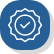平价的专业家庭翻译服务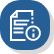全天候待命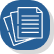我们为您处理一切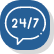操作流程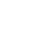确认您的订单

5分钟内在线付款！如果您有任何疑问，您可以电话联系我们，我们将引导您完成整个流程。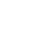接收您的翻译文件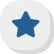您可以信赖的客户服务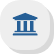具有法律约束力的保密性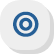推荐和评论

「每当我需要翻译文件，不管是学校的证书、出生证明还是任何其他类型的文件，我都信任西班牙集团能提供高质量的翻译。此外，他们的客服总是愿意帮助我找到最好的选择，并且随时回答我的问题。他们是最好的！」

—安德烈•戈麦斯（得克萨斯休斯顿）

「我第一次使用西班牙集团的服务是为了翻译一份结婚证。我对他们的服务非常满意，现在他们已成了我的翻译服务首选。」

—莱拉•卡特（佛罗里达迈阿密）

立即开始翻译您的文件！

我们的翻译流程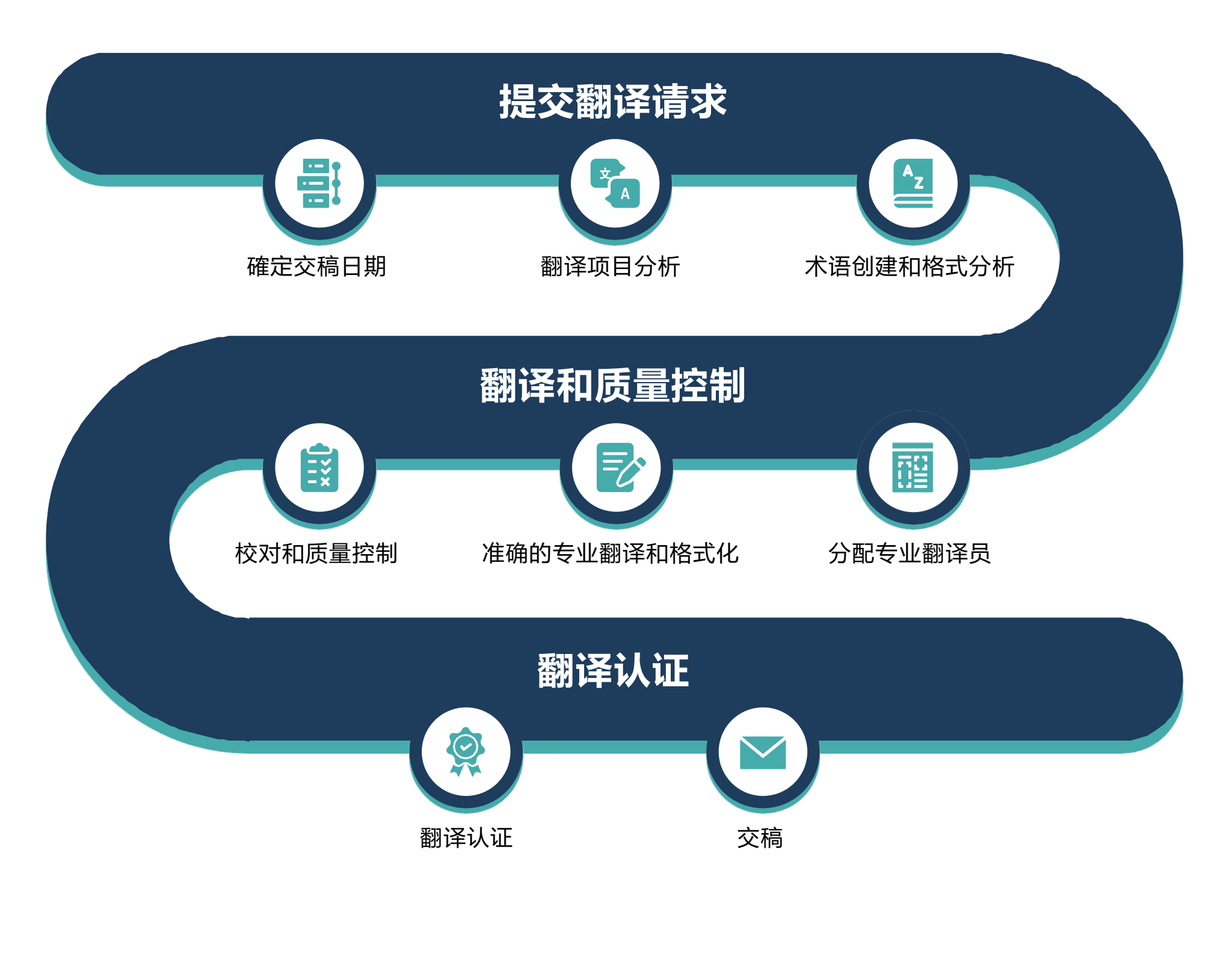认证翻译示例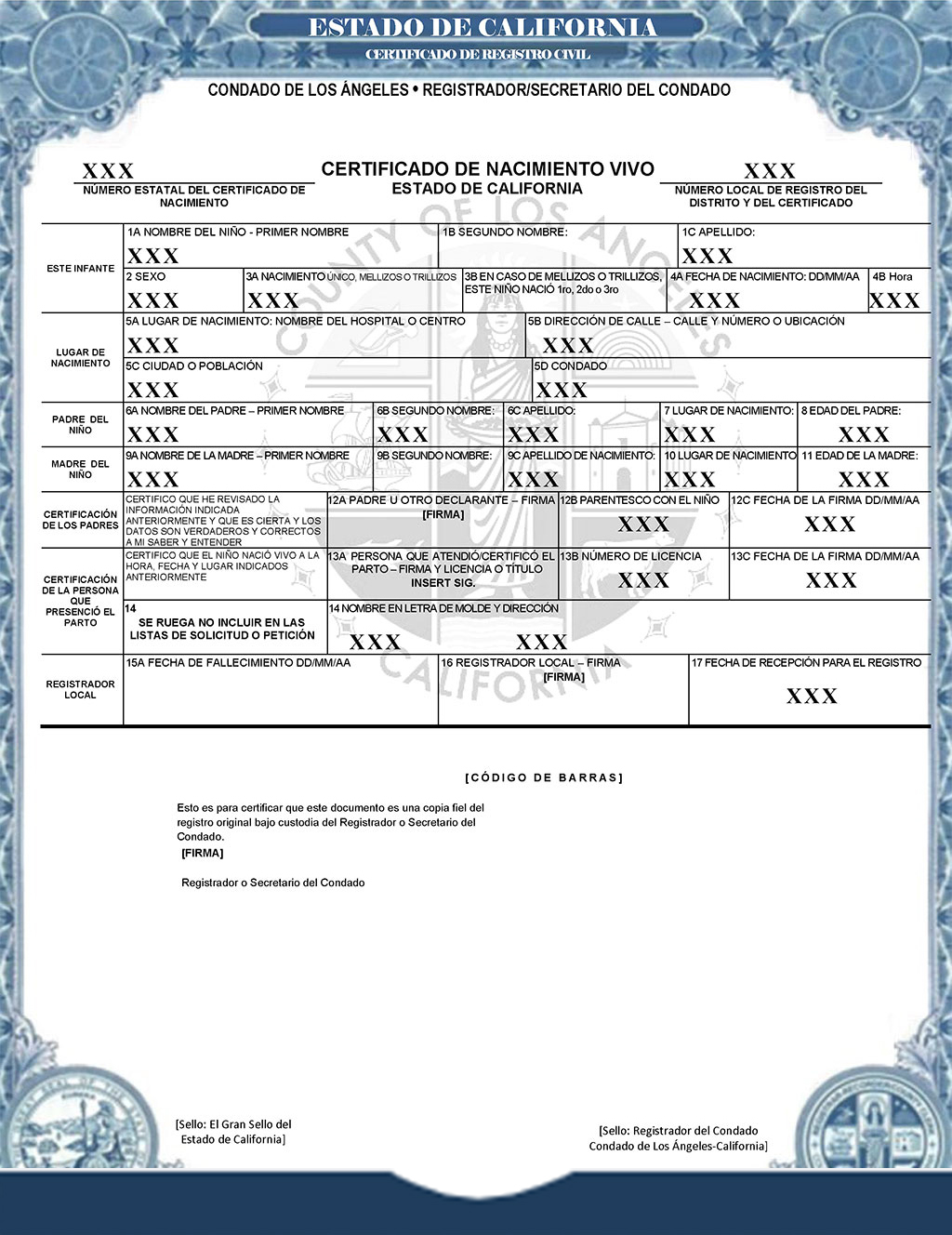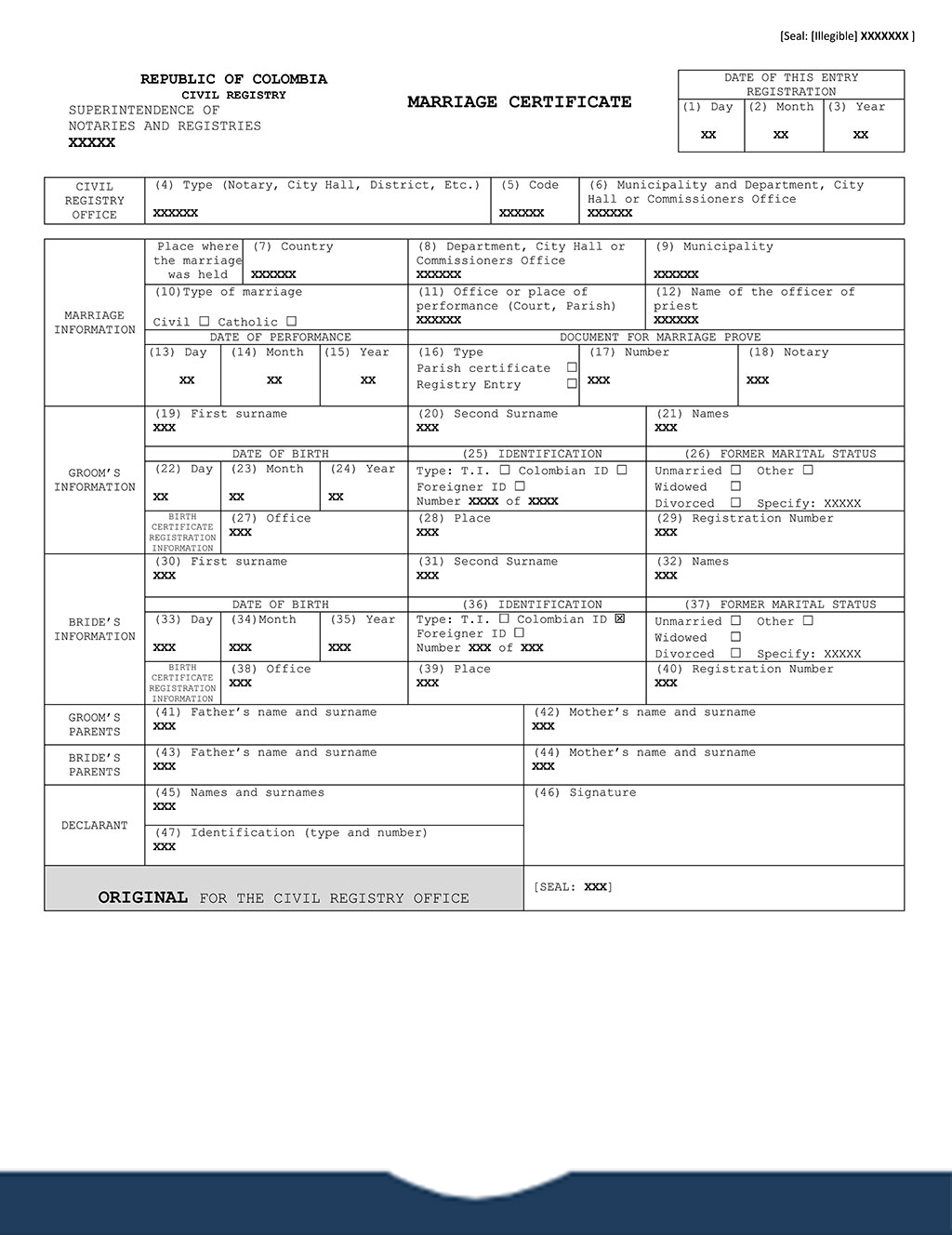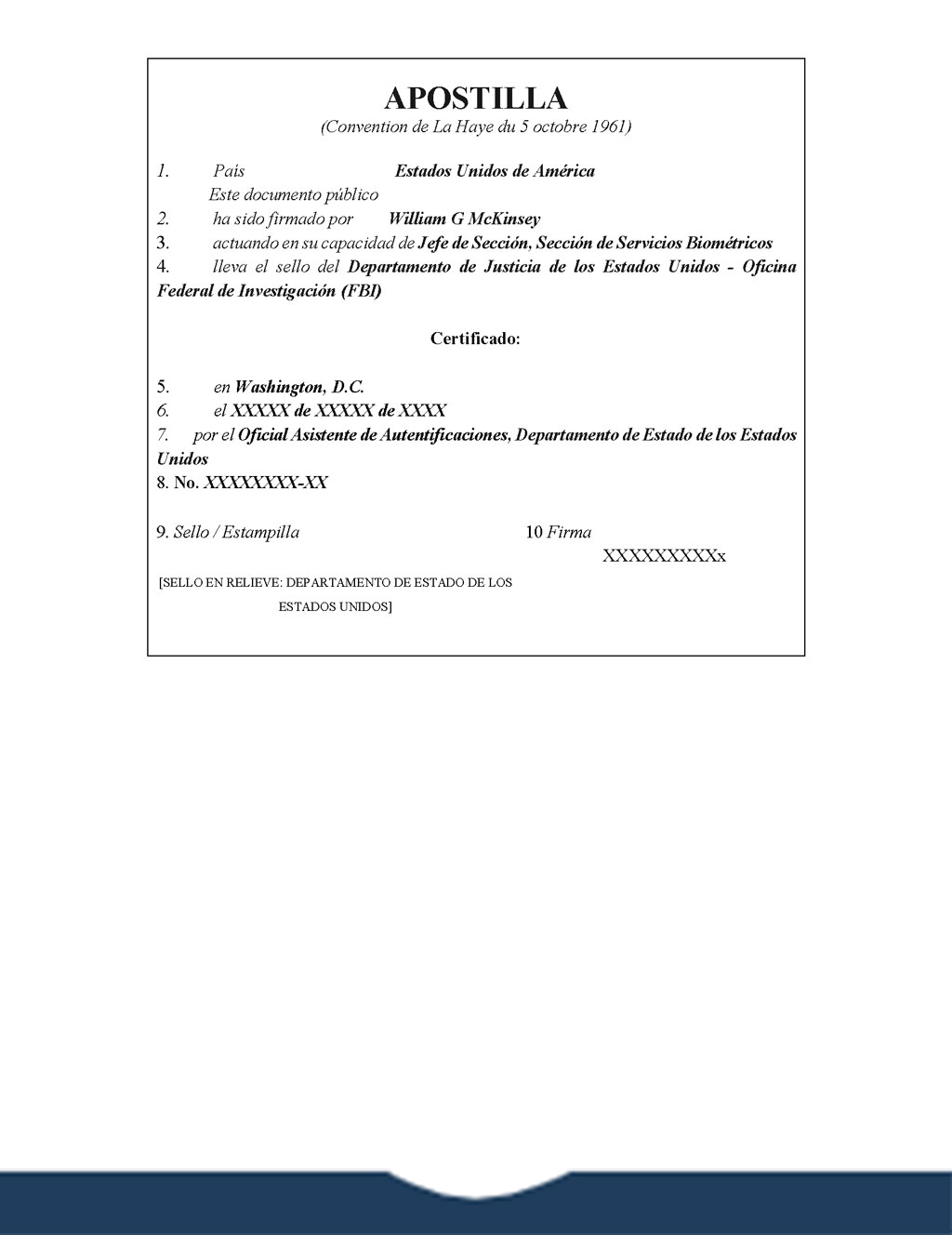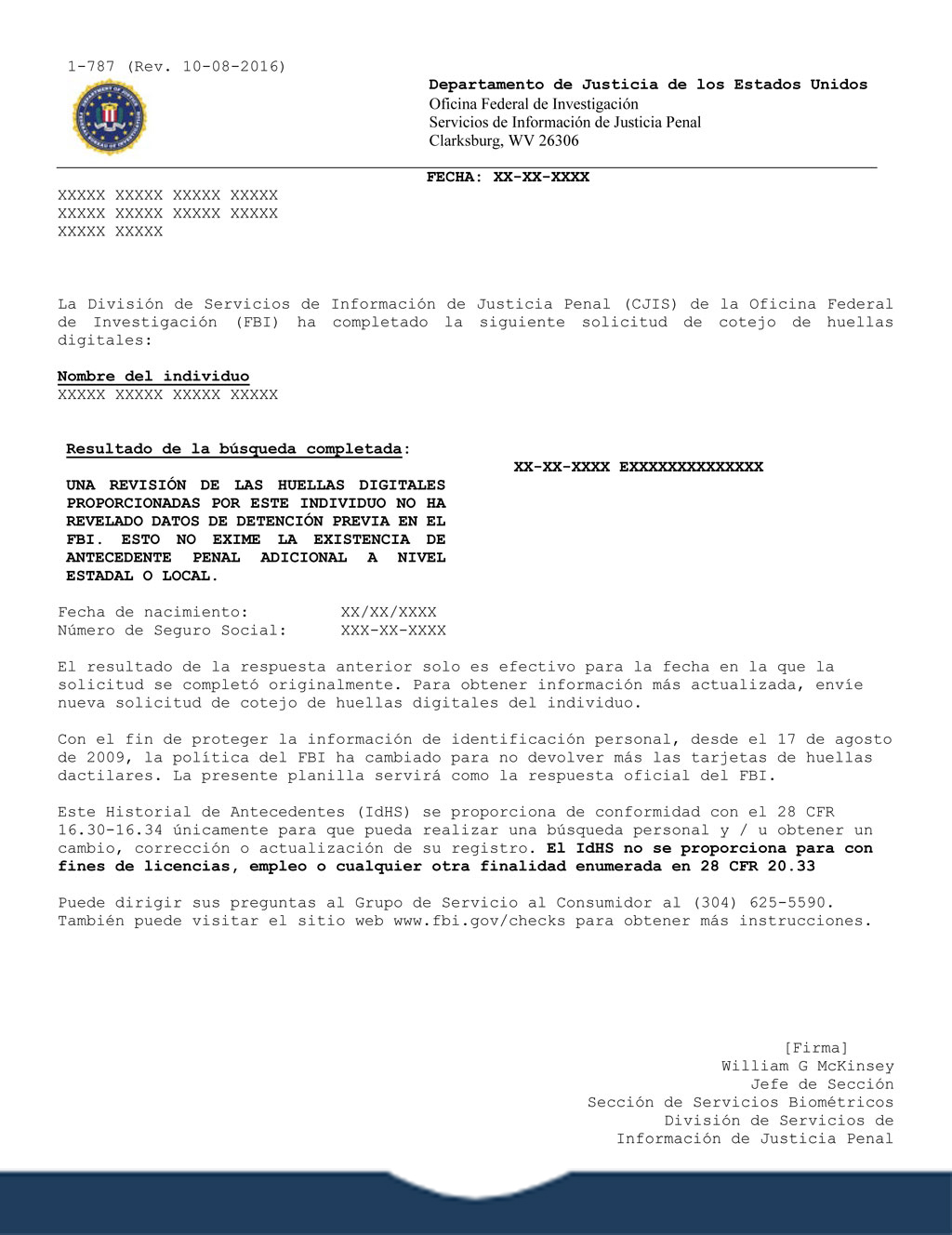常见问题

我们如何帮助您？为什么这么多的家庭选择我们的翻译服务家庭翻译服务涵盖：

•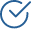平价的按页或按字计价价格
•全天候客户服务
•快速交稿
•高准确性
•包括公证认证*
•完全保密有效性认证国际知名的法律和家庭翻译服务